# Safe Reinforcement Learning Algorithms

## HCOPE (High-Confidence Off-Policy Evaluation.)

Python Implementation of HCOPE lower bound evaluation as given in the paper: Thomas, Philip S., Georgios Theocharous, and Mohammad Ghavamzadeh. "High-Confidence Off-Policy Evaluation." AAAI. 2015.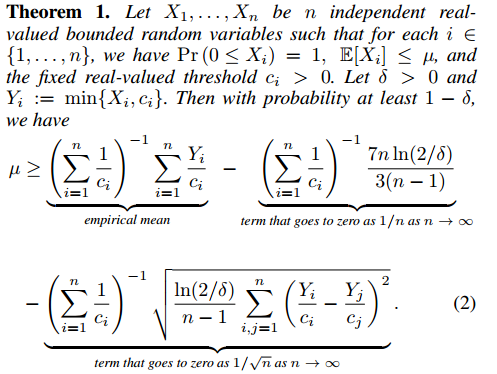• PyTorch
• Numpy
• Matplotlib
• scipy
• gym

### Running Instructions

1. Modify the environment in the main function, choosing from OpenAI gym. (Currently the code works for discrete action spaces)
2. Run python hcope.py

### Notes

• The file policies.py contains the policy used in the code. Modify the policy to suit your needs in this file.
• To reproduce the graph given in the original paper explaining the long tail problem of importance sampling, use the
``````visualize_IS_distribution()
``````

method. Also, a graph of distribution of Importance sampling ratio is created which nicely explains the high variance of the simple IS estimator.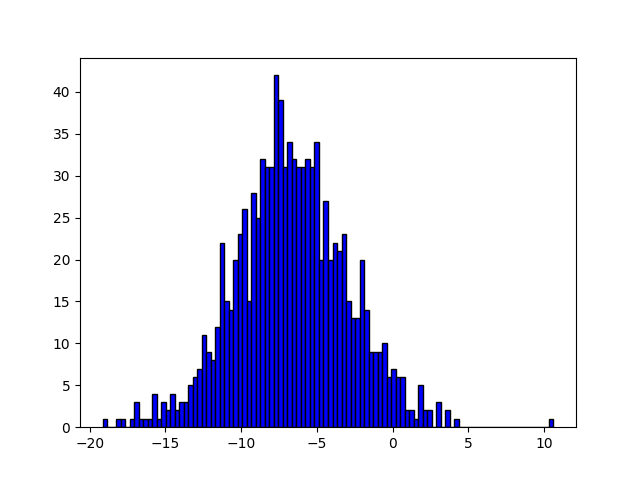• All the values required for offpolicy estimation are initialized in the HCOPE class initialization.

• Currently the estimator policy is defined as a gaussian noise(mean,std_dev) added to the behavior policy for estimator policy initialization in the function `setup_e_policy()`. The example in paper uses policies differing by natural gradient. But, this works as well.

• To estimate c*, I use the BFGS method which does not require computing hessian or first order derivative.

• The `hcope_estimator()` method also implements a sanity check, by computing the discriminant of the quadratic in parameter delta(confidence). If it does not satisfy the basic constraints, the program prints the bound predicted is of zero confidence.

• The random variables are implemented using simple importance sampling. Per-decision importance sampling might lead to better bounds and is to be explored.

• A bilayer MLP policy is used for general problems.

• Results:
Output format: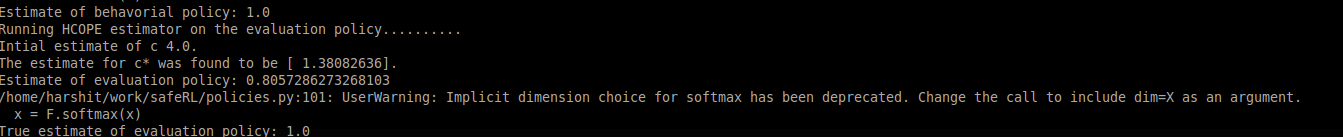## Safe exploration in continuous action spaces.

Paper: Safe Exploration in Continuous Action Spaces - Dalal et al.

### Running Instructions

• Go inside safe_exploration folder
• First learn the safety function by collecting experiences
`python learn_safety_function.py`
• Now using the learned safety function, add the path of these learned torch weights in the train_safe_explorer.py file. After that:
`python train_safe_explorer.py`
This enables agent to learn while following the safety constraints.

### Results

• Safe exploration in a case where constraint is on crossing the right lane marker.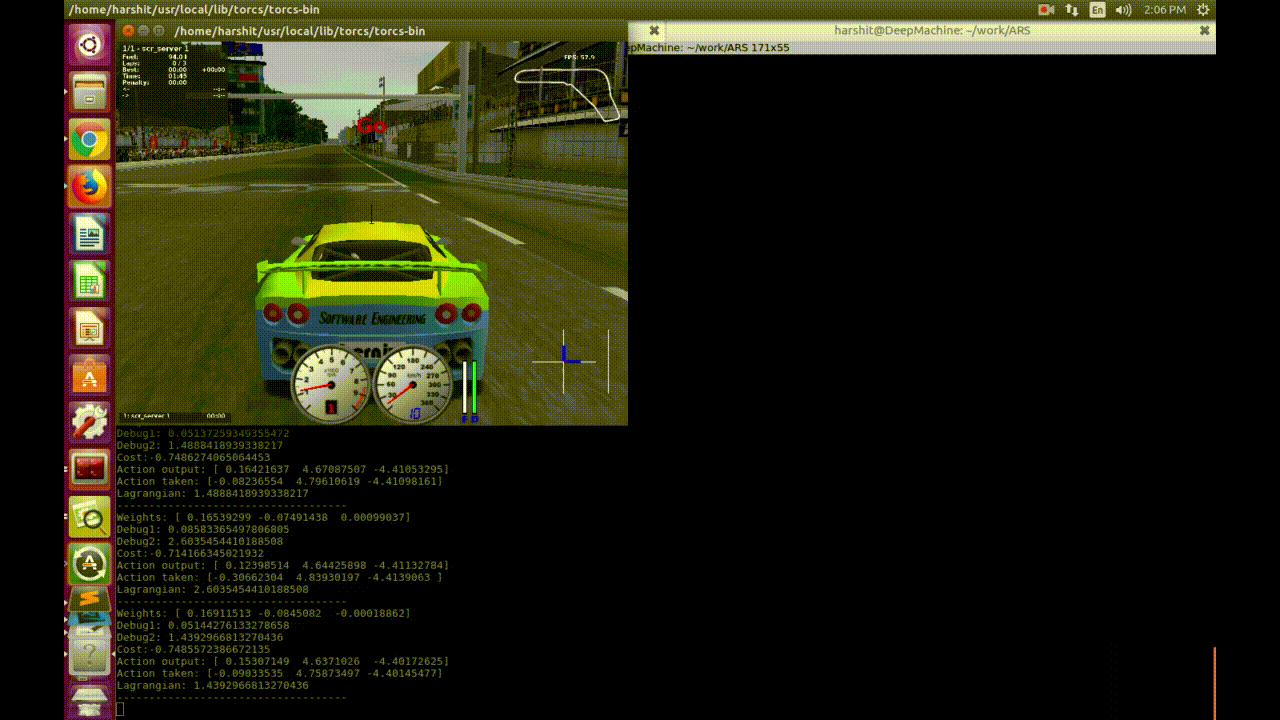• Instability is observed in safe exploration using this method. Here constraint is activated going left through the center of the road.(0.3)### Explanation

• Linear Safety Signal Model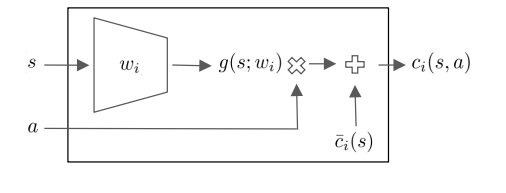• Safety Layer via Analytical Optimization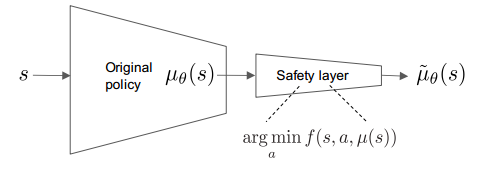• Action Correction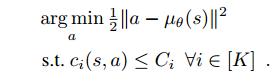## Importance Sampling

Implementation of:

• Simple Importance Sampling
• Per-Decision Importance Sampling
• Normalized Per-Decision Importance Sampling (NPDIS) Estimator
• Weighted Importance Sampling (WIS) Estimator
• Weighted Per-Decision Importance Sampling (WPDIS) Estimator
• Consistent Weighted Per-Decision Importance Sampling (CWPDIS) Estimator

Comparision of different importance sampling estimators: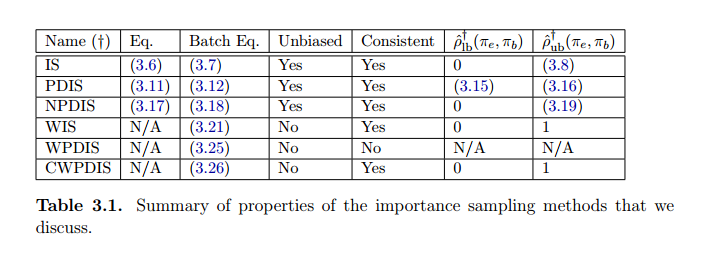Image is taken from phD thesis of P.Thomas:

## Side Effects

### Penalizing side effects using relative reachability

• Added a simple example for calculating side effects as given towards the end of paper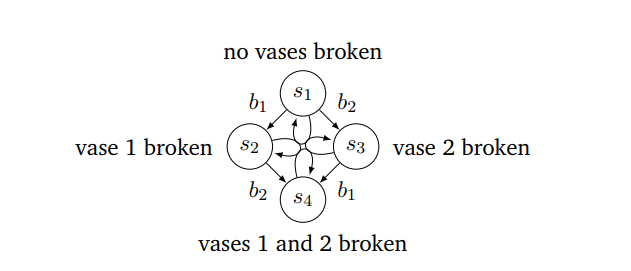The relative reachability measure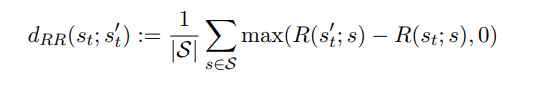Paper: Penalizing side effects using stepwise relative reachability - Krakovna et al.

Get A Weekly Email With Trending Projects For These Topics
No Spam. Unsubscribe easily at any time.
Python (819,784
Reachability (411